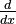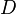# Differential operator facts for kids

Kids Encyclopedia Facts

In mathematics (particularly in differential calculus), a differential operator is an operator that takes a function as input, and returns a function as a result. Differential operators will differentiate the function in one or more variables. Probably the best known differential operator is differentiation itself, which is commonly written using$\tfrac{d}{dx}$ (in Leibniz's notation) and$D$ (in Euler's notation).

## Related pagesDifferential operator Facts for Kids. Kiddle Encyclopedia.